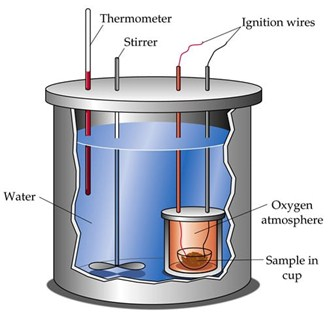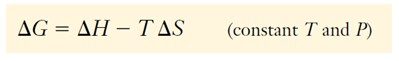Looking for revision notes that are specific to the exam board you are studying? If so, click the links below to view our condensed, easy-to-understand revision notes for each exam board, practice exam question booklets, mindmap visual aids, interactive quizzes, PowerPoint presentations and a library of past papers directly from the exam boards.

# First, Second & Third Law Of Thermodynamics

## Key Information & Summary

• Thermodynamics studies the energy changes in physical and chemical processes.
• Energy is the capacity to do work or to transfer heat.
• Kinetic energy is the energy connected with motion.
• Potential energy is the energy that a system possesses because of its position or composition.
• Reactions that release energy in the form of heat are called exothermic reactions.
• The First Law of Thermodynamics: the total amount of energy in the universe is constant.
• Energy is neither created nor destroyed in ordinary chemical reactions and physical changes.
• The Second Law of Thermodynamics: In spontaneous changes, the universe tends toward a state of greater disorder.
• The Law of Conservation of Energy is just another statement of the First Law of Thermodynamics: Energy is neither created nor destroyed in ordinary chemical reactions and physical changes.
• The thermodynamic state function entropy, S, is a measure of the disorder of the system.
• If G is negative and the process is spontaneous (product-favored).

Thermodynamics studies the energy changes in physical and chemical processes. Usually, these energy changes involve heat this is why “thermo-” is in the word

Energy is the capacity to do work or to transfer heat and it is classified into two general types: kinetic and potential.

Kinetic energy is the energy connected with motion. The kinetic energy of an object is equal to half its mass, m, multiplied by the square of its velocity, v:

Ekinetic  =  1/2mv2

The heavier an object is, the faster it will move, the greater its kinetic energy and the more work it can accomplish.

Potential energy is the energy that a system possesses because of its position or composition.

The work that is necessary to lift an object is stored in the object as potential energy. If the object falls, its potential energy is converted into kinetic energy.

Energy can also be found in many other forms: electrical energy, light, nuclear energy, and chemical energy. At the atomic or molecular level, we can think of each of these as either kinetic or potential energy.

The chemical energy in a fuel or food comes from potential energy stored in atoms due to their arrangements in the molecules. This stored chemical energy can be released when compounds undergo chemical changes, such as those that occur in combustion and metabolism. Reactions that release energy as heat are called exothermic reactions.

Combustion reactions of fossil fuels are familiar examples of exothermic reactions.

Some important ideas about energy are summarized in the First Law of Thermodynamics:

The total amount of energy in the universe is constant.

The Law of Conservation of Energy is just another statement of the First Law of Thermodynamics:

Energy is neither created nor destroyed in ordinary chemical reactions and physical changes.

Most chemical reactions and physical changes occur at constant (usually atmospheric) pressure. The quantity of heat transferred into or out of a system as it undergoes a chemical or physical change at constant pressure, is defined as the enthalpy change, H, of the process. An enthalpy change is sometimes loosely referred to as a heat change or a heat of reaction.
The enthalpy change is equal to the enthalpy or “heat content,” H, of the substances produced, minus the enthalpy of the substances consumed.It is impossible to know the absolute enthalpy (heat content) of a system. Enthalpy is a state function, however, and it is the change in enthalpy in which we are interesting; this can be measured for many processes. We can determine the energy change associated with a chemical or physical process by using an experimental technique called calorimetry. This technique is based on observing the temperature change when a system absorbs or releases energy in the form of heat.

The experiment is carried out in a device called a calorimeter, in which the temperature change of a known amount of substance (often water) of known specific heat is measured. The temperature change is caused by the absorption or release of heat by the chemical or physical process under study.The thermodynamic standard state of a substance is its most stable pure form, under standard pressure (one atmosphere) and at some specific temperature (25°C or 298 K unless otherwise specified). Some examples of elements in their standard states at 25°C are hydrogen, gaseous diatomic molecules, H2(g), mercury, a silver-colored liquid metal.

For a gas, the standard state is the gas at a pressure of one atmosphere; in a mixture of gases, its partial pressure must be one atmosphere. For a substance in solution, the standard state refers to one-molar concentration.

The standard enthalpy change, H0, refers to the H when reactants, all at standard states, are converted completely to products, all at standard states.

The standard molar enthalpy of formation, Hf 0 , of a substance is the enthalpy change involved when one mole of the substance is formed from its elements in their standard states. By convention, the Hf 0 value for any element in its standard state is zero.

Enthalpy is a state function. Its change is therefore independent of the pathway by which a reaction occurs.

Energies can help understanding if a reaction will be spontaneous or not. As instance spontaneity is favoured when heat is released during the change (exothermic). Furthermore, a reaction is more likely to happen if it causes an increase in disorder.

The Second Law of Thermodynamics helps in this:

In spontaneous changes, the universe tends toward a state of greater disorder.

When a mirror is dropped, it can shatter. When a drop of wine is added to a glass of water, it mixes until a homogeneous solution is reached.

The thermodynamic state function entropy, S, is a measure of the disorder of the system. The greater the disorder of a system, the higher is its entropy. The entropy of any substance increases as the substance goes from solid to liquid to gas because the particles are more highly ordered in the solid state than in the liquid state, thank in the gas.

The Third Law of Thermodynamics establishes the zero of the entropy scale.

The entropy of a pure, perfect crystalline substance (perfectly ordered) is zero at absolute zero (0 K).

As the temperature of a substance increases, the particles vibrate more vigorously, so the entropy increases. Further heat input causes either increased temperature (still higher entropy) or phase transitions (melting, sublimation, or boiling) that also result in higher entropy. The entropy of a substance at any condition is its absolute entropy, also called standard molar entropy.

The relationship between enthalpy and entropy was formulated by J. Willard Gibbs (1839–1903), a prominent nineteenth-century American professor of mathematics and physics.

Gibbs free energy, G is a state function and the Gibbs free energy change, G, at constant temperature and pressure, isThe amount by which the Gibbs free energy decreases is the maximum useful energy obtainable in the form of work from a given process at constant temperature and pressure. It is also the indicator of spontaneity of a reaction or physical change at constant T and P. If G is negative and the process is spontaneous (product-favored).